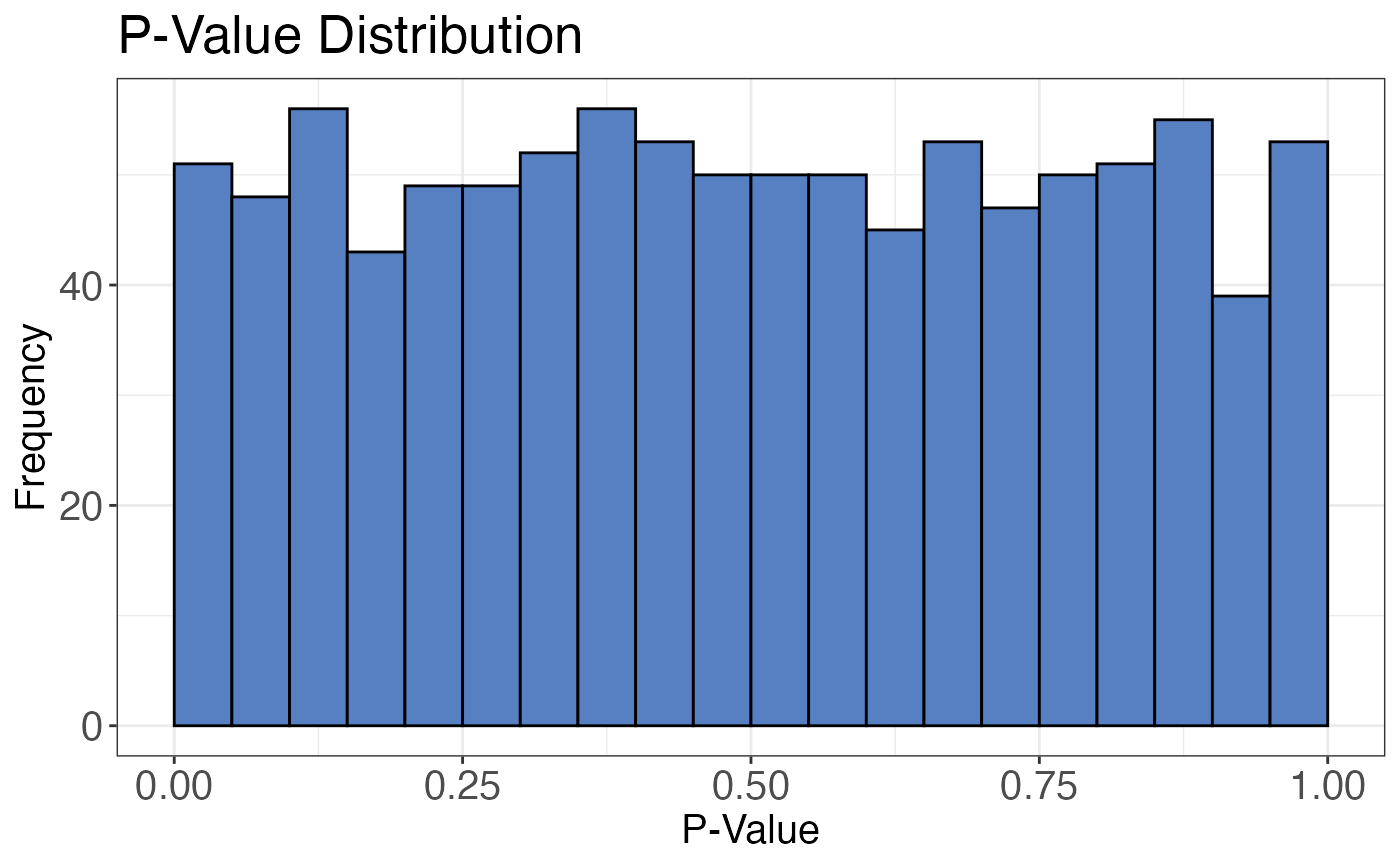Plots the distribution of p-values derived from any statistical test as a histogram.

pval_distribution_plot(data, grouping, pval)

## Arguments

data a data frame that contains at least grouping identifiers (precursor, peptide or protein) and p-values derived from any statistical test. a character column in the data data frame that contains either precursor, peptide or protein identifiers. For each entry in this column there should be one unique p-value. That means the statistical test that created the p-value should have been performed on the level of the content of this column a numeric column in the data data frame that contains p-values.

## Value

A histogram that shows the p-value distribution.

## Examples

set.seed(123) # Makes example reproducible

# Create example data
data <- data.frame(
peptide = paste0("peptide", 1:1000),
pval = runif(n = 1000)
)

# Plot p-values
pval_distribution_plot(
data = data,
grouping = peptide,
pval = pval
)